Photonics Research, 2023, 11 (9): 1570, Published Online: Aug. 28, 2023

### Optical leaky fin waveguide for long-range optical antennas on high-index contrast photonic circuit platforms

1 Photonics Research Group, Department of Information Technology, Ghent University - IMEC, Ghent, Belgium
2 Center for Nano- and Biophotonics (NB Photonics), Ghent University, Ghent, Belgium
Figures & Tables

#### Fig. 1. 3D concept drawing of the proposed antenna structure. A vertical fin on top of a rectangular waveguide can couple the light upward depending on the position of this fin. The radiation strength is proportional to the asymmetry of the structure, defined by the offset of the fin from the center position of the waveguide core. The fin has a strong uniform grating on top to couple the light from the fin to free space, but this grating remains constant (no apodization) throughout the entire antenna design. The core layer tapers underneath the fin structure to maintain the propagation constant of the optical mode, required for emitting a Gaussian beam with a flat phase front. The simulations in this work are carried out with SiN as core and fin material, but the principle also works for Si platforms.#### Fig. 2. (a) Conceptual illustration of a solid-state LiDAR system integrated on a chip surface. An integrated 1D OPA with dispersive antennas is used in combination with a tunable laser to limit the number of input waveguides and phase shifters to N. A simplified ranging engine is also illustrated, where a frequency modulation approach can be implemented by using the same laser as a local oscillator and creating a beating frequency with the FM-modulated signal received back from the OPA. This approach is referred to as swept-source LiDAR . The feeding network of these dispersive antennas resembles traditional Butler  or Nolen  networks from microwave systems. The feeding network can be constructed with a feed-forward Mach–Zehnder mesh circuit [17,18]. (b) To have a large enough Rayleigh range, the near-field beam diameter 2w0 should be large enough, both in the x and y directions (illustrated with the “x/y” label on the vertical axis). In other words, the field divergence θdiv should be small enough for an adequate resolution [δθx and δθy, see Fig. 3(a)].#### Fig. 3. (a) Illustration of an integrated 1D OPA with dispersive antennas on the surface of a chip, consisting of a splitter tree, an array of electro-optic phase shifters connected to an emitting surface, which consists of a dense array of long (in the y direction) (apodized) grating coupler antennas. Important parameters such as the divergence angle θdiv, the related resolution δθx,δθy, and the field of view Δθx,Δθy are visualized. Increasing the aperture size Ly to 3w0 improves the performance. (b) Detailed illustration of the dispersive antenna elements in the 1D OPA implemented as long apodized grating couplers. The pitch between the emitters (gratings) (Px) should be sufficiently small, for which an HIC is required (enables a high density of antenna elements in the OPA). This implies a very high in-plane aspect ratio of the antenna elements, which makes it difficult to implement a weak grating. The effect of apodization of the grating strength is emphasized by illustrating the undesired exponential decay of an unapodized (i.e., constant parameters along y) grating. The weak part at the beginning of the grating is the most challenging and demanding part of the fabrication, but also the most crucial for a good beam profile.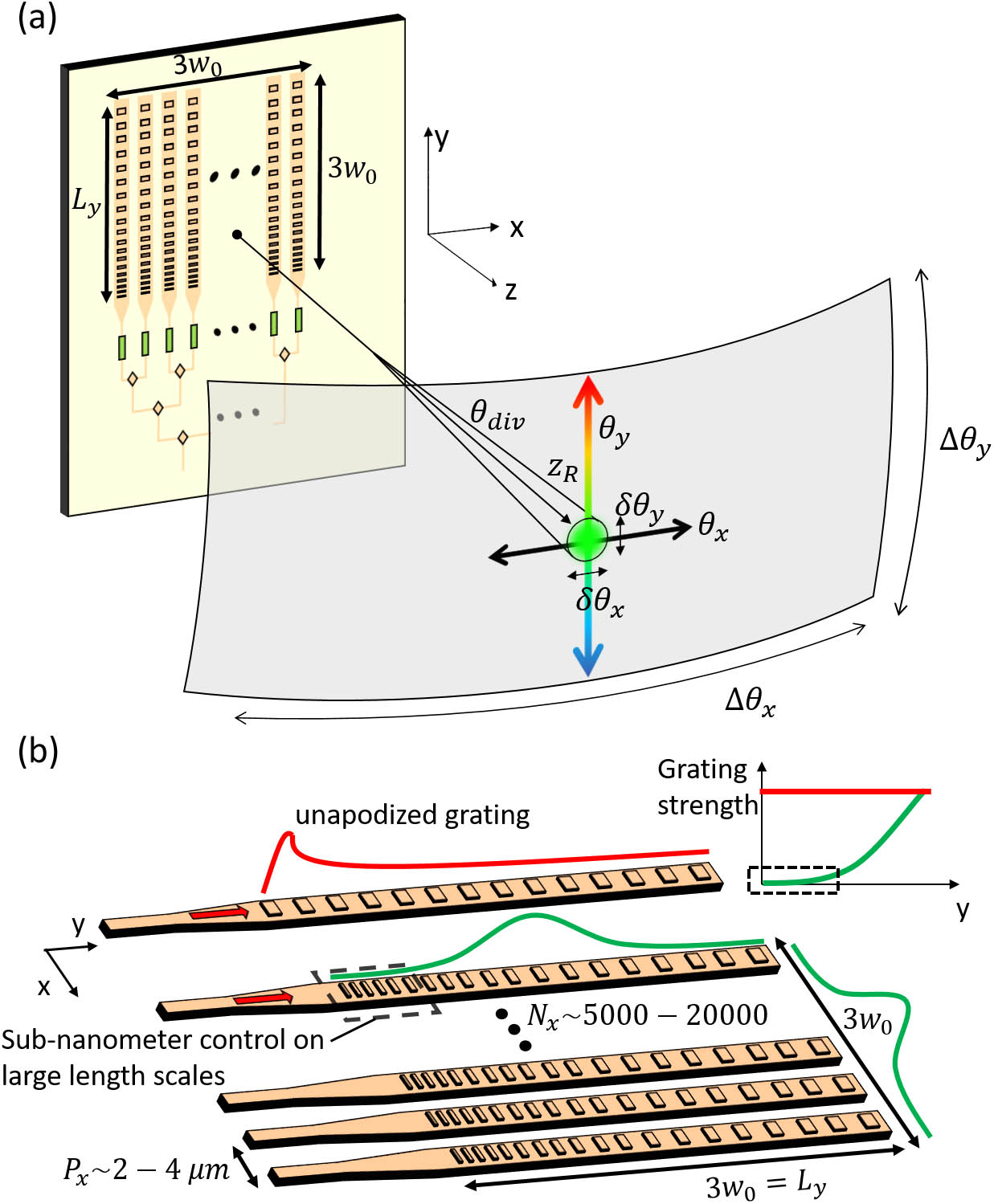#### Fig. 4. Illustration of the film mode matching modeling configuration of the vertical leaking structure. The top and bottom boundary condition is implemented as a transparent boundary condition (TBC), while the sides are implemented as perfect electric conductors (PECs). The structure is analyzed for different values of the waveguide core width wcore and the offset. The core thickness, tcore, and the width of the fin wfin have the same value for all simulations. The transparent boundary condition on the top surface is equivalent to continuing the physical fin toward infinity, guiding power outside the simulation window at the radiation angle θy. Simulations in this work use SiN as core and fin material, but the principle also works for Si platforms.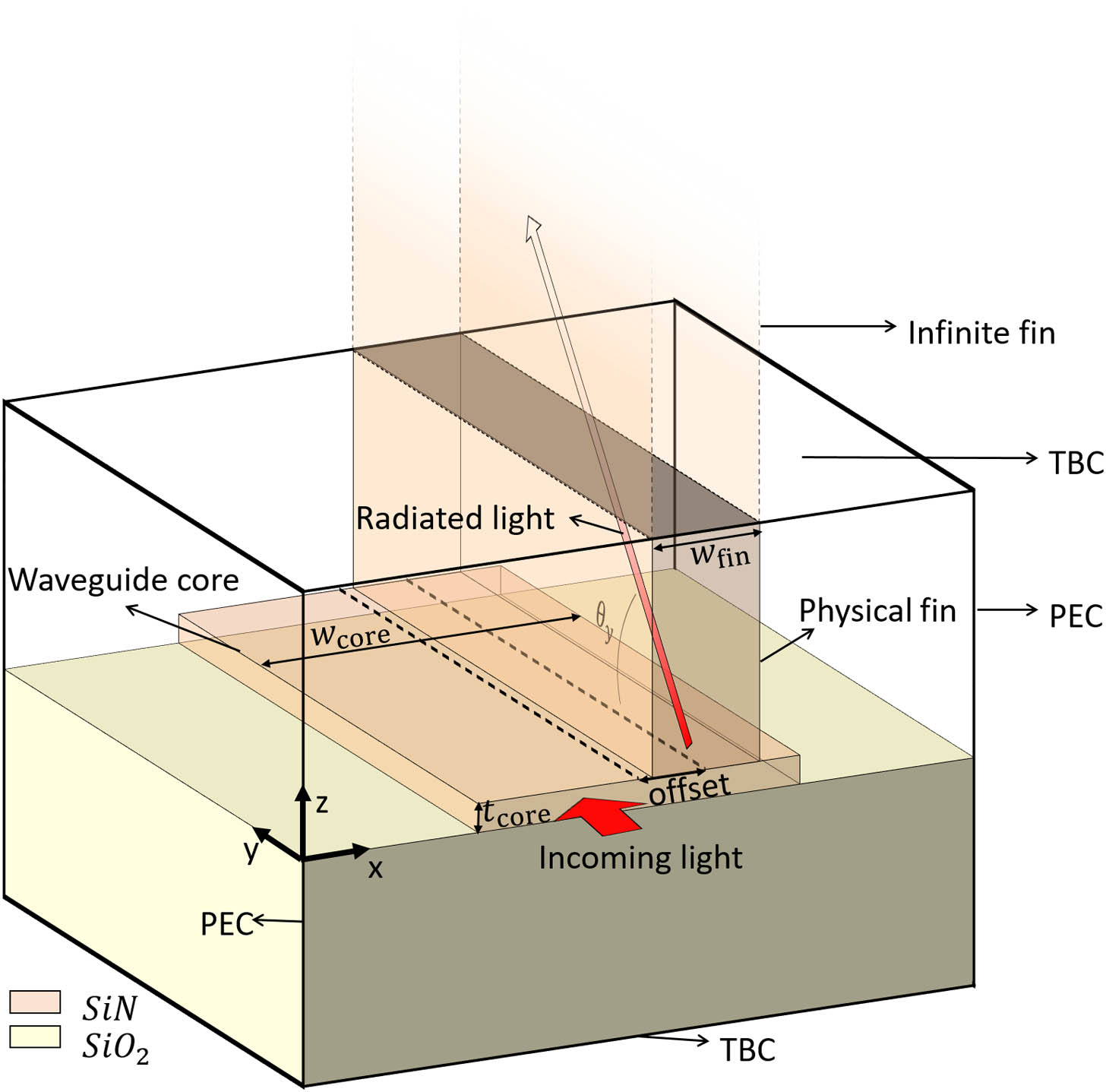#### Fig. 5. Radiation strength α of the cross section in dB/cm, plotted with iso-n contours visualized. For a given constant phase constant β of the antenna, design values have to be selected from one iso-n contour. For these simulations λ=1.55  μm, tcore=300  nm, and wfin=400  nm.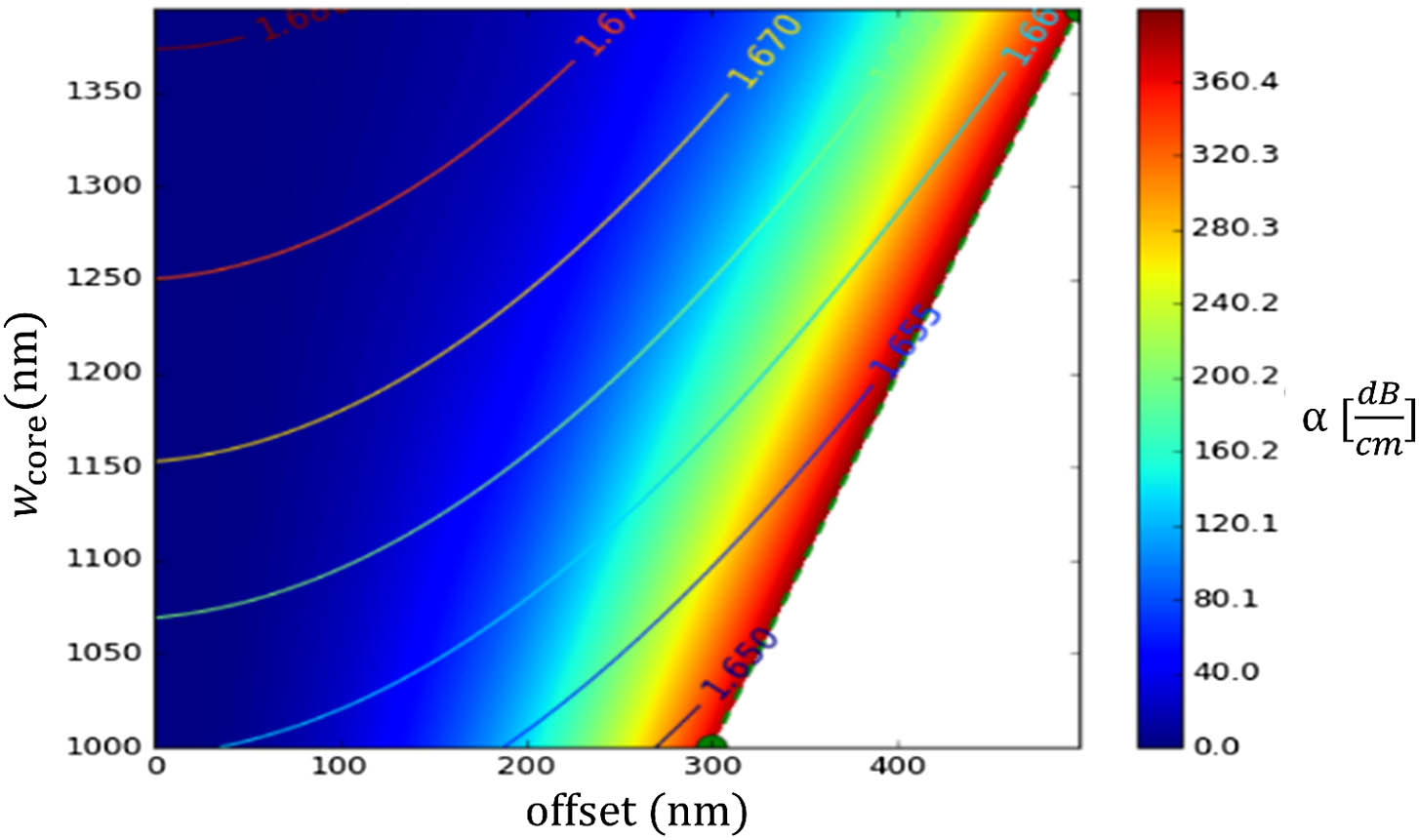#### Fig. 6. Imaginary part of longitudinal field component ℑ{Ey}. (a) Zero offset (lossless symmetric structure). (b) Maximum symmetry breaking by increasing the offset to the maximum value. The red curves show ℑ{Ey} sampled on the aperture of the vertical fin (the top surface of the waveguide) indicated in the figure with a white dotted line. Breaking the symmetry of the structure results in an increasing overlap with the slab mode of the fin because the mode profile becomes highly asymmetric in the waveguide.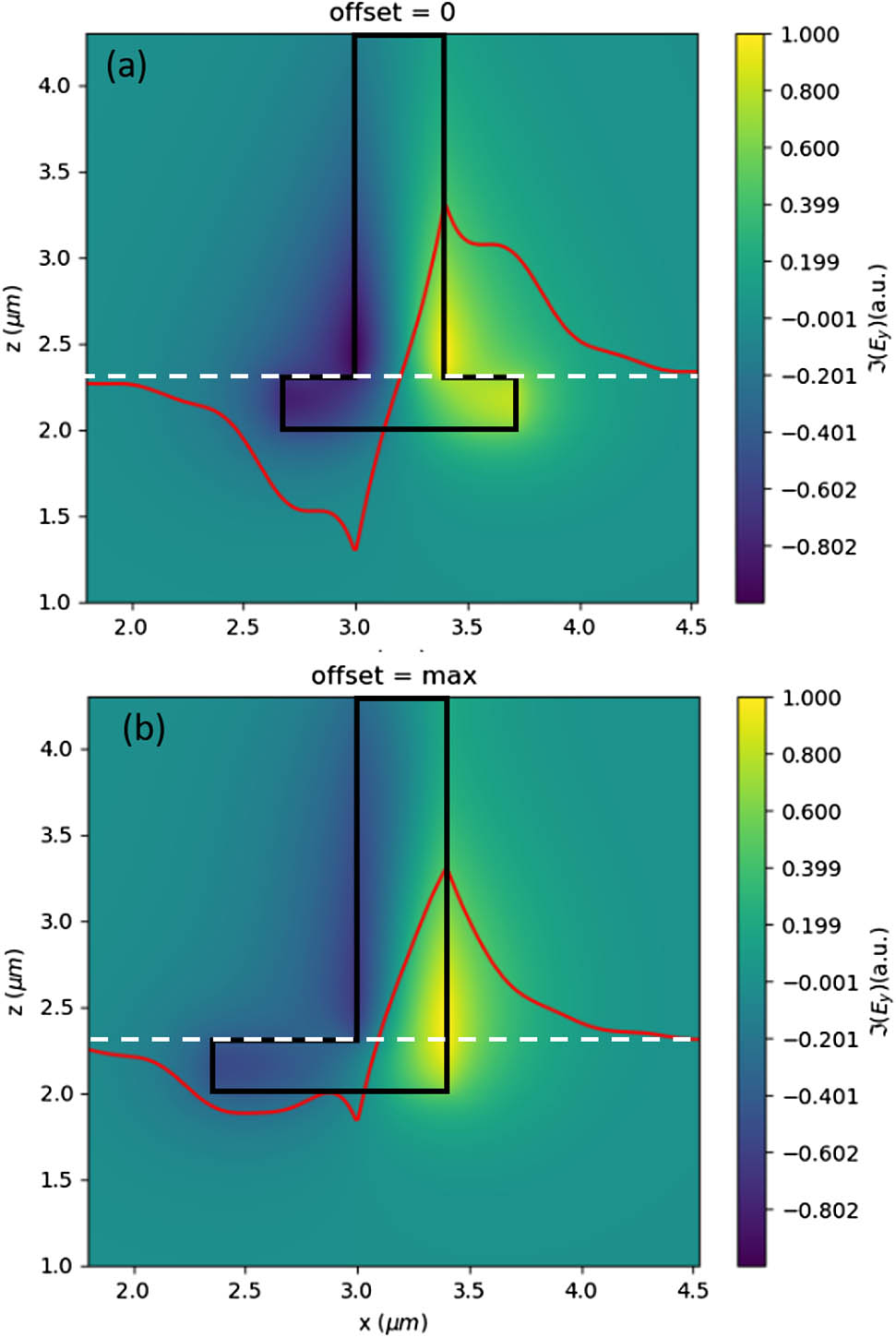#### Fig. 7. Values of the partial derivatives from the spline interpolation used to create Fig. 5 to determine a prediction of the sensitivity to fabrication variation in both wcore and the offset. The iso-n contour with n≈1.662 is highlighted in each plot. (a) Variation of n with the offset in 10−6nm. (b) Variation of n with the width in 10−6nm. (c) Variation of α with the offset in dB/cmnm. (d) Variation of α with the width in dB/cmnm.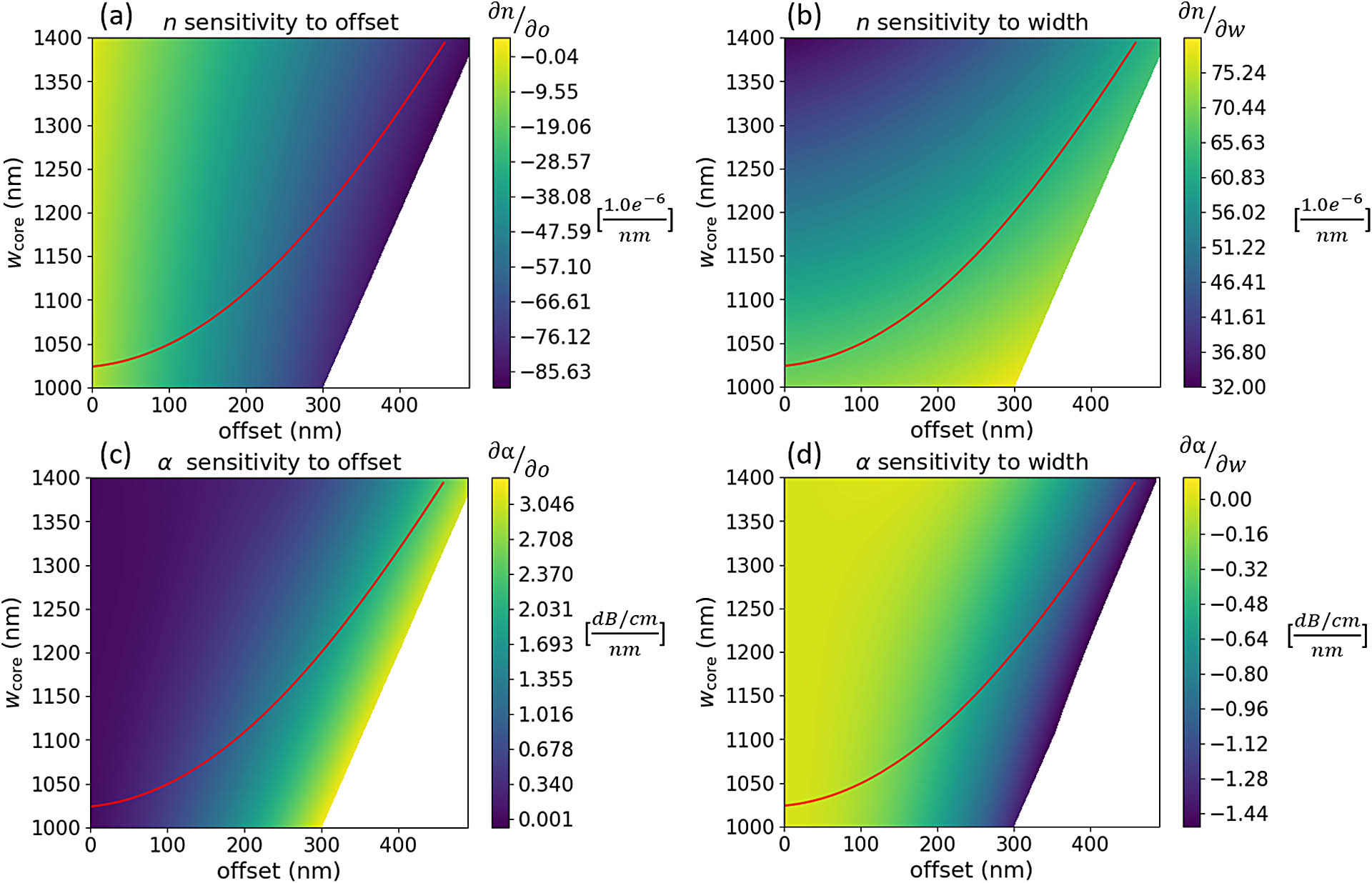#### Fig. 8. Corresponding n values with increasing offset following the n-contour for the antenna design with λ=1.55  μm. Ideally, all values remain constant with increasing offset, but at wavelength deviating from the design wavelength, we see a deviation of the initial value of n when the offset increases. The deviation is stronger at higher values of the offset.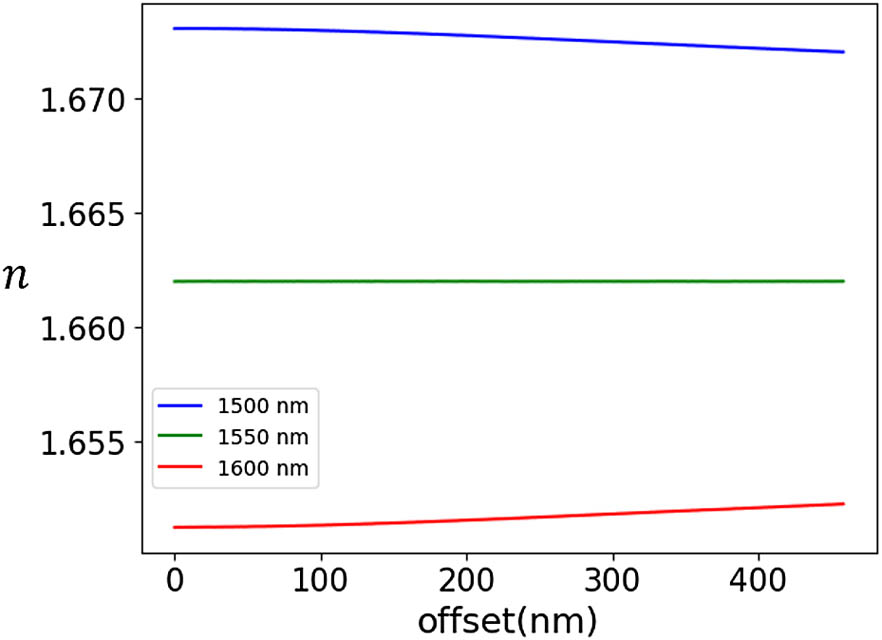#### Fig. 9. Discretization of the antenna in segments to calculate required α from radn for each segment. By requiring PN=0, meaning that all incoming (normalized) power P0 is radiated, the entire radiation profile can be back-calculated.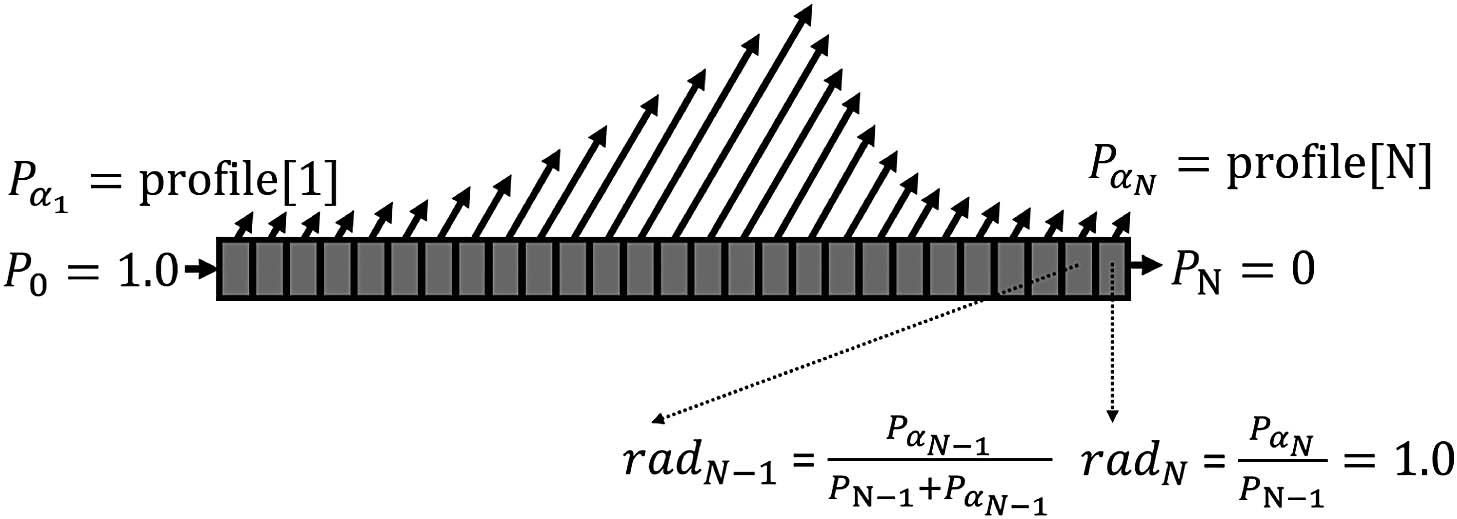#### Fig. 10. (a) Target Gaussian power profile (w0=8  mm) for antenna, centered at 15 mm. This profile function is sampled with a step of 100 μm, so each point profile[n] resembles the power emitted by 1 segment of the discretization. (b) Output α(y) of the back-calculation illustrated in Fig. 9. This function is used to determine the cross-sectional parameters at each y coordinate.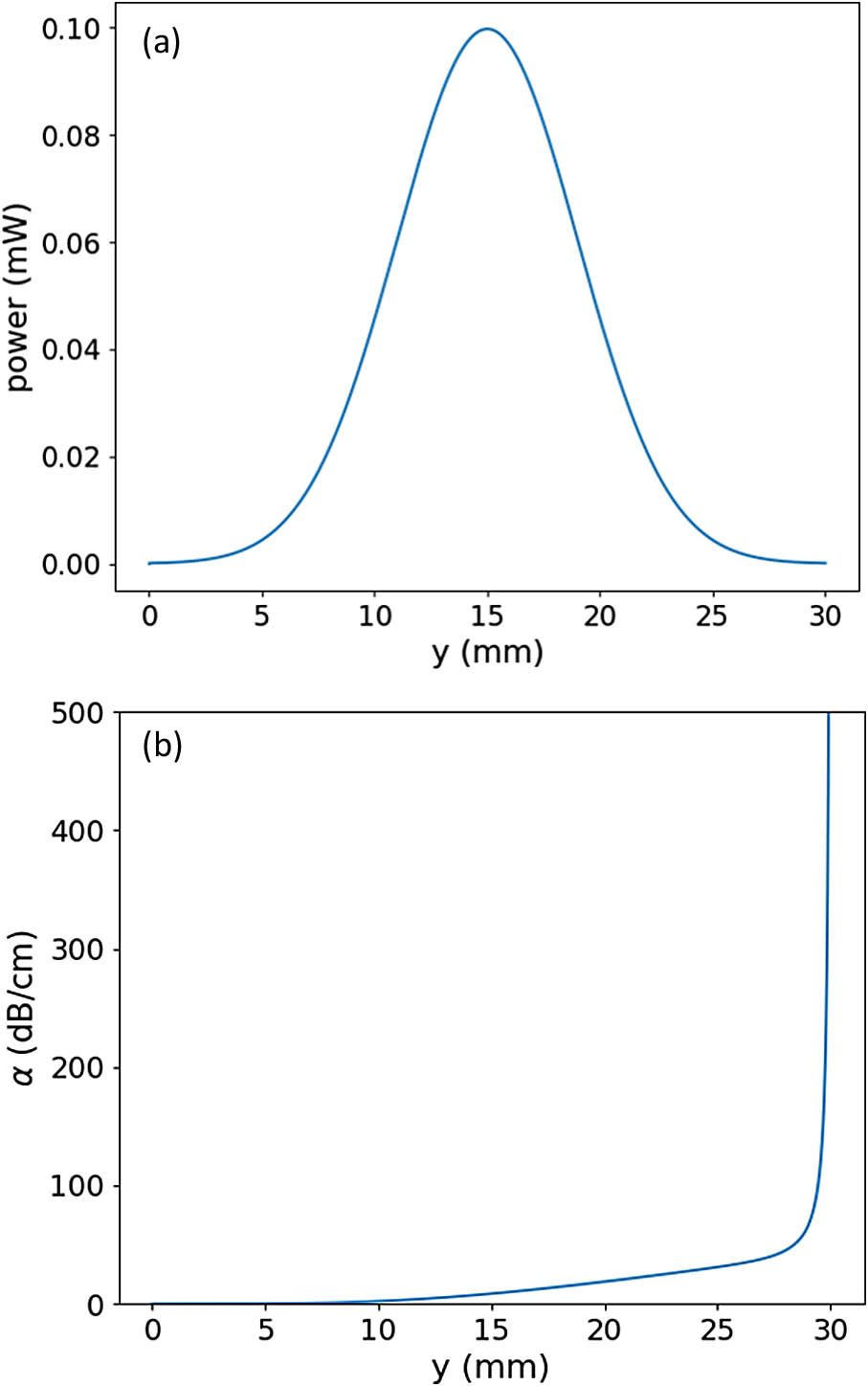#### Fig. 11. (a) Fitted polynomial wcore(offset) to sampled points on iso-n-contour with n=1.662 (λ=1.55  μm) from Fig. 5. (b) Fit of offset to corresponding α values on sampled iso-n-contour, offset(α). If α(y) from Fig. 10(b) is used as an input, with (b) we can determine the required offset at each y position, after which we can determine the required width with (a) to ensure constant β.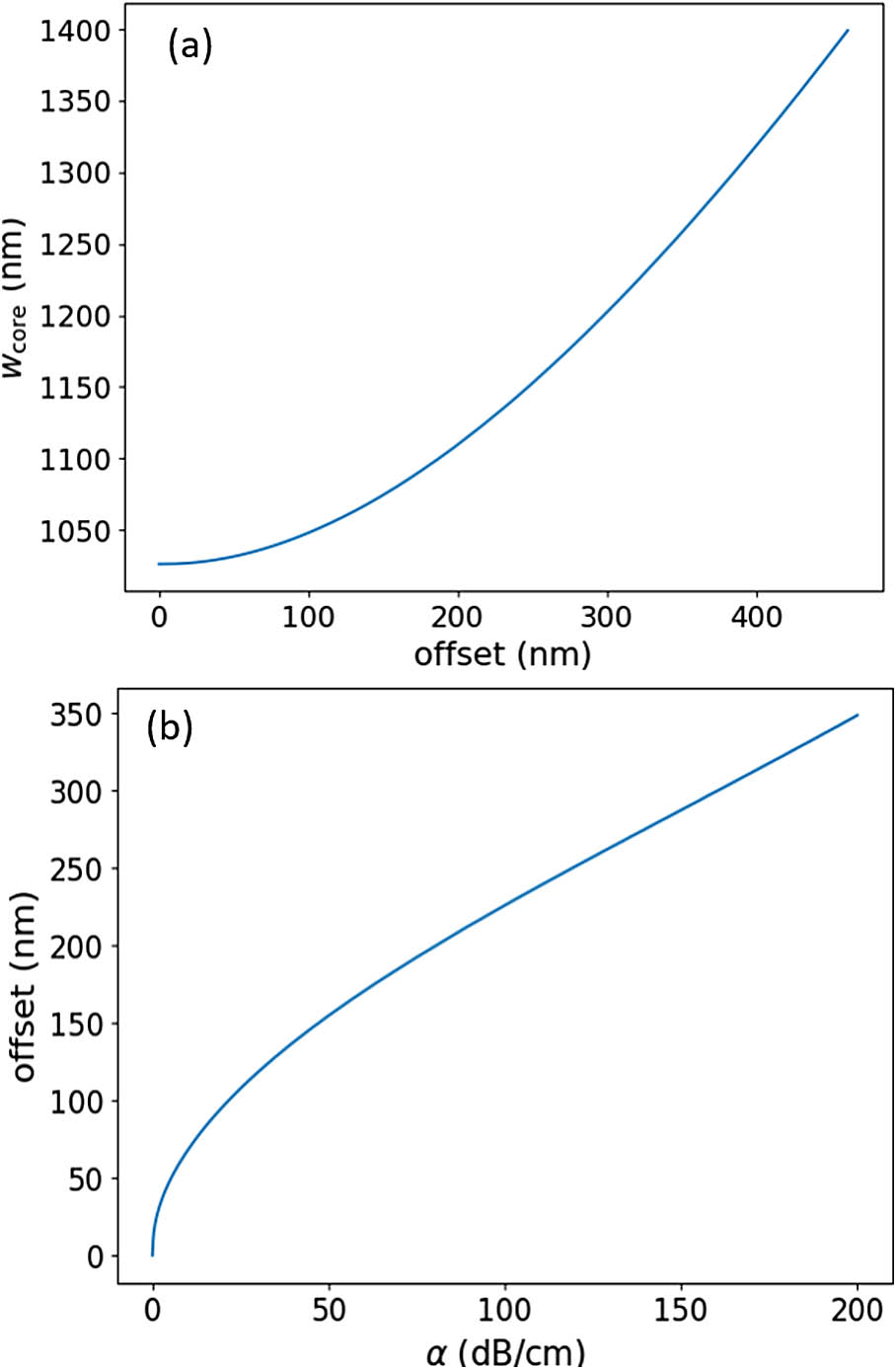#### Fig. 12. (a) Resulting tapering function wcore(y) from Fig. 11(a) using offset(y) as input. (b) Resulting offset function offset(y) from Fig. 11(b) starting from target function Fig. 10(b). The small bump is caused by numerical errors in the bi-variate fit near the low-loss regions because the loss increases very gradually with the offset for small values.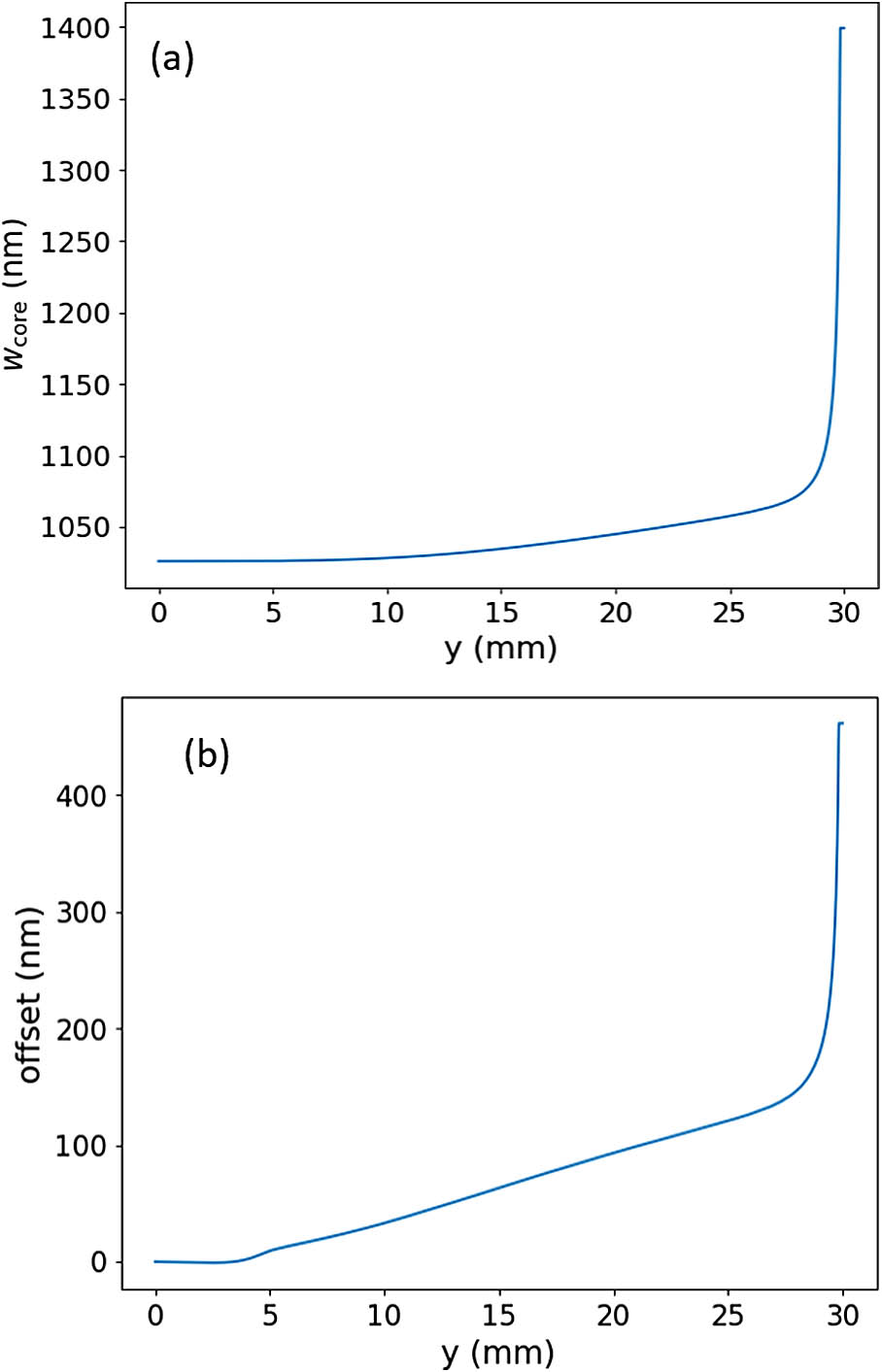#### Fig. 13. Top view of an array of the proposed antenna geometry, which now replaces the fabrication sensitive gratings used in Figs. 2 and 3. Note that the high aspect ratio of the antenna is required for dense integration along the x axis.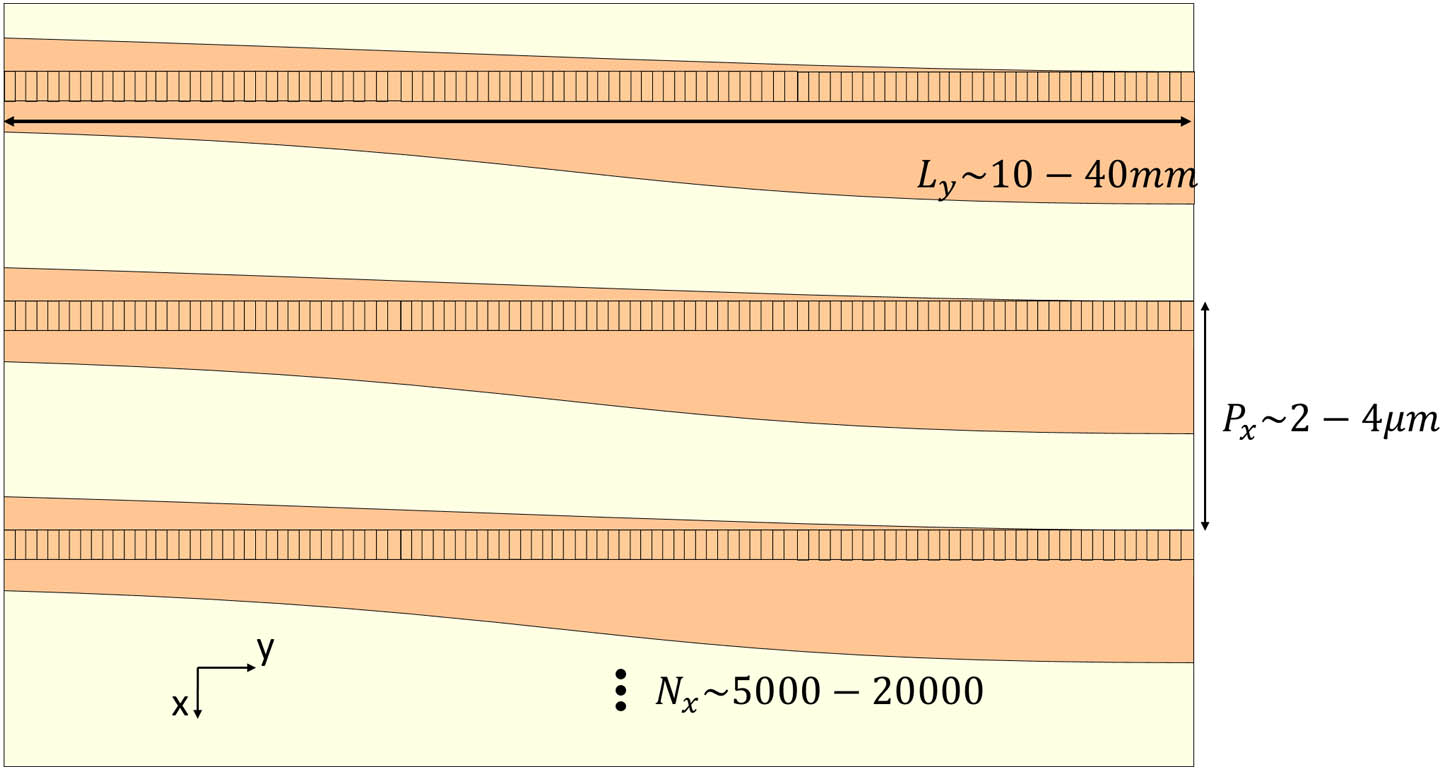#### Fig. 14. (a) Top view of a 3D simulation result of the antenna design (λ=1.55  μm) presented in Section 5. In this simulation, segments with a length of 100 μm were used to discretize the design, and then FMM with transparent boundary conditions was used to determine the modes of each segment. The core layer and fin are visualized to aid the interpretation of the field plot. Note the high aspect ratio of the simulation window. (b) 1D view of the power on the black dotted line, together with the target Gaussian [Fig. 10(a)]. Due to the discretization, a small ripple is introduced on the simulated power profile. The bump caused by numerical errors is also visible here.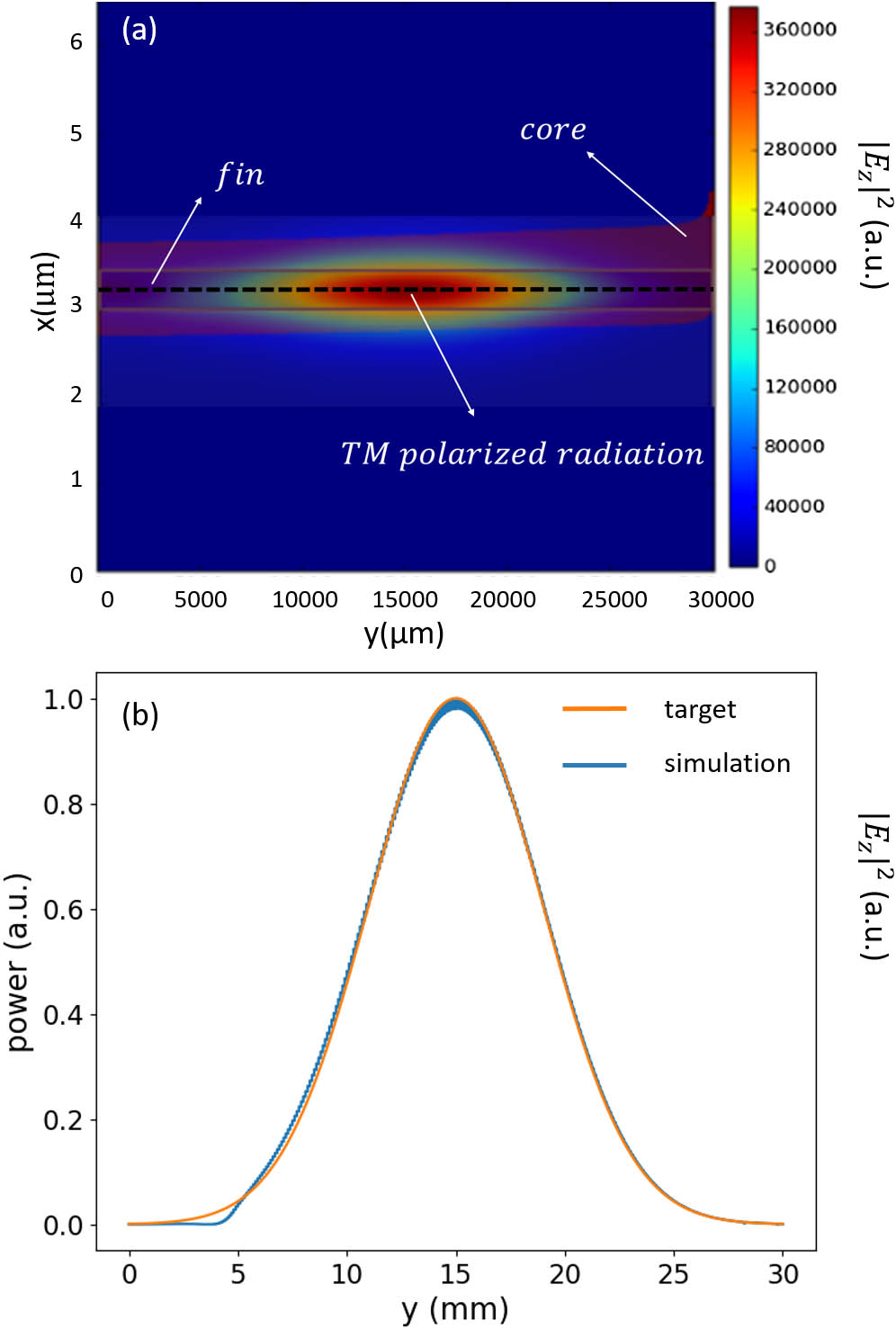#### Fig. 15. (a) Calculated far field at λ=1.55  μm from simulated E field in the aperture, compared to the ideal target far field (target Gaussian with perfect phase ramp). (b) Simulated wavelength dependence of far-field angle. The FWHM value is indicated for each wavelength to assess the wavelength dependence of the divergence.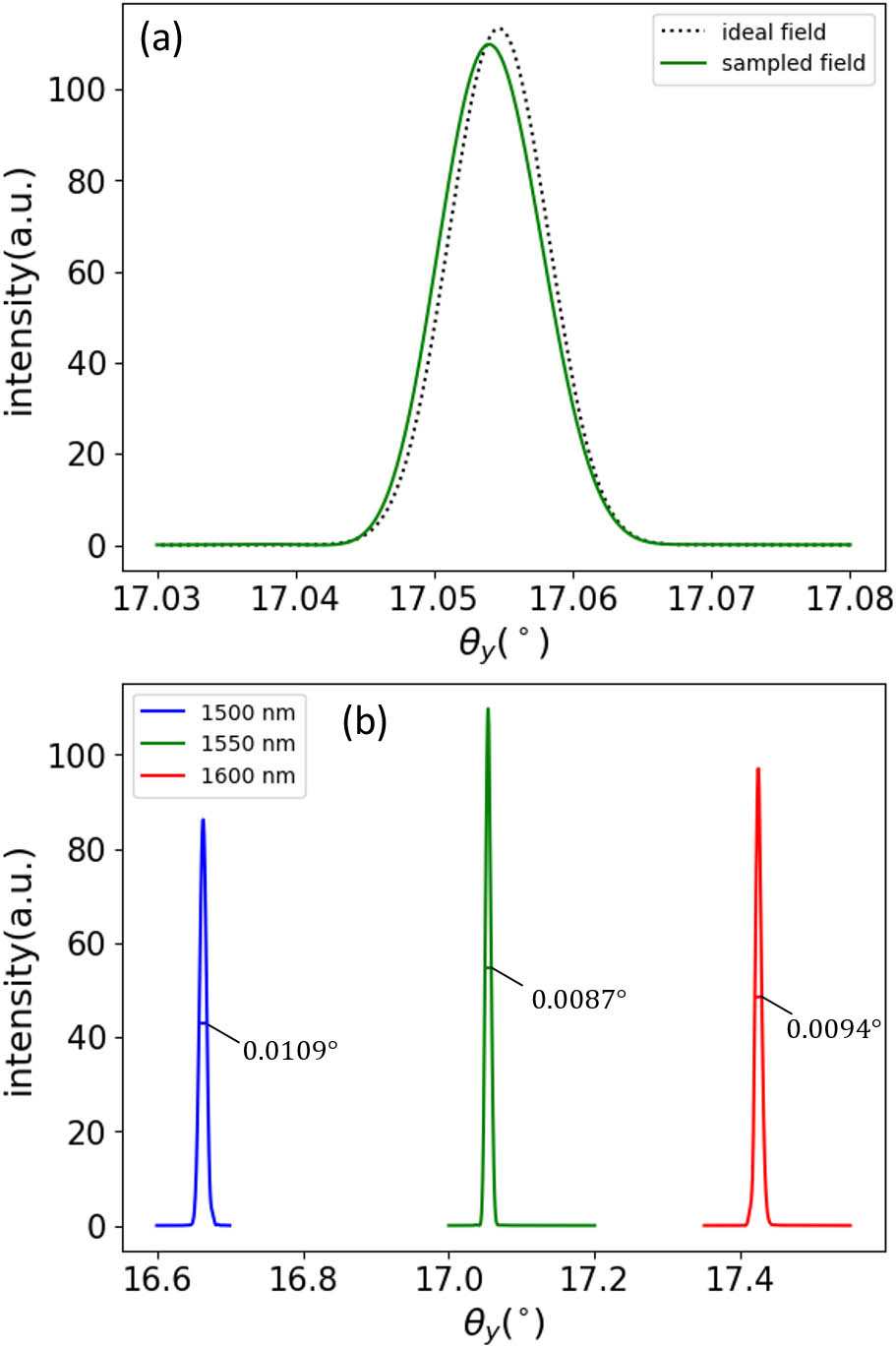Lukas Van Iseghem, Wim Bogaerts. Optical leaky fin waveguide for long-range optical antennas on high-index contrast photonic circuit platforms[J]. Photonics Research, 2023, 11(9): 1570.